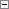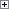Returns the total number of elements in an array or the number of elements in a specific dimension.

Length := ARRAYLEN(Array[, Dimension])

Parameters

Array

Type: Any

The array that you want to investigate.

Dimension

Type: Integer

If you omit this optional argument, the function returns the total number of elements in the array. To get the number of elements in a specific dimension, use Dimension with a valid value. The valid values are determined by the number of dimensions of the input array. For example, the valid values for a three-dimensional array would be 1, 2, and 3.Property Value/Return Value

Type: Integer

The number of elements in the array, or the number of elements in a specific dimension.Remarks

If you use ARRAYLEN with an input parameter that is not an array, a run-time error occurs.Example

This example shows how to use the ARRAYLEN function.

This example requires that you create the following variables in the C/AL Globals window.

Variable name DataType Dimension

Array1

Integer

2

Array2

Integer

3;4Copy Code
MESSAGE('Array1, Total number of elements: %1', ARRAYLEN(Array1));
MESSAGE('Array2, Dimension 1 size: %1', ARRAYLEN(Array2,1));
MESSAGE('Array2, Dimension 2 size: %1', ARRAYLEN(Array2,2));
MESSAGE('Array2, Total number of elements: %1', ARRAYLEN(Array2));

The following messages are displayed.

Array1, Total number of elements: 2

Array2, Dimension 1 size: 3

Array2, Dimension 2 size: 4

Array2, Total number of elements: 12